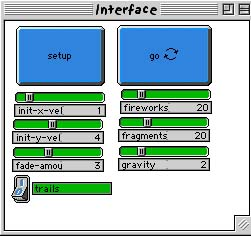WHAT IS IT?
-----------
This program models the action of fireworks.  A rocket begins at the
bottom of the screen, shoots upwards into the sky and then explodes,
emitting a shower of descending sparks.  Each rocket, represented by a
turtle, is launched upward with an initial x and y velocity.  At a
certain point in the sky, an explosion occurs, which is represented by a
series of turtle hatches.  Each hatched turtle inherits the velocity from
the original rocket in addition to velocity from the explosion itself.
The result is a simulation of a fireworks display.

HOW TO USE IT
--------------
SETUP sets up the model according to the values indicated by all the
sliders and the switch.
GO is a forever button that executes the model continually.  FIREWORKS
creates a random number of fireworks between 0 and the number indicated
on the slider.
FRAGMENTS determines how many particle fragments will emerge after the
explosion of a single firework.
GRAVITY determines the gravitational strength in the environment.  A
larger value will give a greater gravitational acceleration, meaning that
particles will be forced to the ground at a faster rate.  The inverse is
true for smaller values.
INIT-X-VEL sets the initial x-velocity of each rocket to a random number
between the negative and positive value of the number indicated on the
slider.
INIT-Y-VEL sets the initial y-velocity of each rocket to a random number
between 0 and the number indicated on the slider plus ten.  This is to
ensure that there is a range of difference in the initial y-velocities of
the fireworks.
after the explosiion.

TRAILS allows the user to turn the trails left by the explosion particles
on or off.  In other words, if the TRAILS switch is on 1, then the
turtles will leave trails.  If it is on 0, then they will not leave
trails.

If the GO button is stopped and started again after the model begins, all
the particles from an explosion explode again.  This is because the model
is treating each particle as a rocket.  Notice also that the particles in
the second explosion inherit the velocities from the particles in the
first.

This model has been constructed so that all changes in the sliders and
switches will take effect in the model during execution.  So, while the
GO button is still down, you can change the values of the sliders and the
switch, and you can see these changes being represented on the graphics
window.

THINGS TO NOTICE
----------------
Experiment with the INIT-X-VEL and INIT-Y-VEL sliders.  Observe that at
an initial x-velocity of zero, the rockets launch straight upwards.  When
the initial x-velocity is increased, notice that some rockets make an arc
to the left or right in the sky depending on whether the initial
x-velocity is negative or positive.

With the initial y-velocity, observe that, on a fixed GRAVITY value, the
heights of the fireworks are lower on smaller initial y-velocities and
higher on larger ones.  Also observe that each rocket explodes at a
height equal to or a little less than its apex.

THINGS TO TRY
-------------
Observe what happens to the model when the GRAVITY slider is set to
different values.  Watch what happens to the model when GRAVITY is set to
zero.  Can you explain what happens to the fireworks in the model?  Can
you explain why this phenonmenon occurs?  What does this say about the
importance of gravity?  Now set the GRAVITY slider to its highest value.
What is different about the behavior of the fireworks at this setting?
What can you conclude about the relationship between gravity and how
objects move in space?

EXTENDING THE MODEL
-------------------
The fireworks represented in this model are only of one basic type.  A
good way of extending this model would be to create other more complex
kinds of fireworks.  Some could have multiple explosions, multiple
colors, or a specific shape engineered into their design.

Notice that this model portrays fireworks in a two-dimensional viewpoint.
When we see real fireworks, however, they appear to take a
three-dimensional form.  Try extending this model by converting its
viewpoint from 2D to 3D.

StarLogoT FEATURES
-----------------
An important aspect of this model is the fact that each particle from an
explosion inherits the properties of the original firework.  This
informational inheritance allows the model to adequately represent the
projectile motion of the firework particles since their initial x and y
velocities are relative to their parent firework.

To visually represent the fading property of the firework particles, this
model made use of the turtle command scale-color.  As the turtle
particles fall to the ground, they hold their pens down and gradually
scale their color to black.  As mentioned above, the rate of fade CAN be